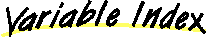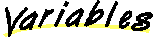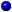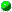``` All Packages Class Hierarchy This Package Previous Next Index```

# Class java.lang.Double

``` java.lang.Object | +----java.lang.Number | +----java.lang.Double ```
public final class Double
extends Number
The Double class wraps a value of the primitive type `double` in an object. An object of type `Double` contains a single field whose type is `double`.

In addition, this class provides several methods for converting a `double` to a `String` and a `String` to a `double`, as well as other constants and methods useful when dealing with a `double`.

##MAX_VALUE
The largest positive value of type `double`.MIN_VALUE
The smallest positive value of type `double`.NaN
A NaN value of type `double`.NEGATIVE_INFINITY
The negative infinity of type `double`.POSITIVE_INFINITY
The positive infinity of type `double`.TYPE
The Class object representing the primitive type double.

##Double(double)
Constructs a newly allocated `Double` object that represents the primitive `double` argument.Double(String)
Constructs a newly allocated `Double` object that represents the floating- point value of type `double` represented by the string.

##byteValue()
Returns the value of this Double as a byte (by casting to a byte).doubleToLongBits(double)
Returns a representation of the specified floating-point value according to the IEEE 754 floating-point "double format" bit layout.doubleValue()
Returns the double value of this Double.equals(Object)
Compares this object against the specified object.floatValue()
Returns the float value of this Double.hashCode()
Returns a hashcode for this Double.intValue()
Returns the integer value of this Double (by casting to an int).isInfinite()
Returns true if this Double value is infinitely large in magnitude.isInfinite(double)
Returns true if the specified number is infinitely large in magnitude.isNaN()
Returns true if this Double value is the special Not-a-Number (NaN) value.isNaN(double)
Returns true if the specified number is the special Not-a-Number (NaN) value.longBitsToDouble(long)
Returns the double-float corresponding to a given bit represention.longValue()
Returns the long value of this Double (by casting to a long).shortValue()
Returns the value of this Double as a short (by casting to a short).toString()
Returns a String representation of this Double object.toString(double)
Creates a string representation of the `double` argument.valueOf(String)
Returns a new Double value initialized to the value represented by the specified String.

##POSITIVE_INFINITY ``` public static final double POSITIVE_INFINITY ```
The positive infinity of type `double`.NEGATIVE_INFINITY ``` public static final double NEGATIVE_INFINITY ```
The negative infinity of type `double`.NaN ``` public static final double NaN ```
A NaN value of type `double`.MAX_VALUE ``` public static final double MAX_VALUE ```
The largest positive value of type `double`.MIN_VALUE ``` public static final double MIN_VALUE ```
The smallest positive value of type `double`.TYPE ``` public static final Class TYPE ```
The Class object representing the primitive type double.

##Double ``` public Double(double value) ```
Constructs a newly allocated `Double` object that represents the primitive `double` argument.

Parameters:
value - the value to be represented by the `Double`.Double ``` public Double(String s) throws NumberFormatException ```
Constructs a newly allocated `Double` object that represents the floating- point value of type `double` represented by the string. The string is converted to a `double` value as if by the `valueOf` method.

Parameters:
s - a string to be converted to a `Double`.
Throws: NumberFormatException
if the string does not contain a parsable number.
valueOf

##toString ``` public static String toString(double d) ```
Creates a string representation of the `double` argument.

The values `NaN`, `NEGATIVE_INFINITY`, `POSITIVE_INFINITY`, `-0.0`, and `+0.0` are represented by the strings `"NaN"`, `"-Infinity"`, `"Infinity"`, `"-0.0"`, and `"0.0"`, respectively.

If `d` is in the range `10-3 <= |d| <=107`, then it is converted to a string in the style `[-]ddd.ddd`. Otherwise, it is converted to a string in the style `[-]m.ddddE±xx`.

There is always a minimum of one digit after the decimal point. The number of digits is the minimum needed to uniquely distinguish the argument value from adjacent values of type `double`.

Parameters:
d - the double to be converted.
Returns:
a string representation of the argument.valueOf ``` public static Double valueOf(String s) throws NumberFormatException ```
Returns a new Double value initialized to the value represented by the specified String.

Parameters:
s - the string to be parsed.
Returns:
a newly constructed `Double` initialized to the value represented by the string argument.
Throws: NumberFormatException
if the string does not contain a parsable number.isNaN ``` public static boolean isNaN(double v) ```
Returns true if the specified number is the special Not-a-Number (NaN) value.

Parameters:
v - the value to be tested.
Returns:
`true` if the value of the argument is NaN; `false` otherwise.isInfinite ``` public static boolean isInfinite(double v) ```
Returns true if the specified number is infinitely large in magnitude.

Parameters:
v - the value to be tested.
Returns:
`true` if the value of the argument is positive infinity or negative infinity; `false` otherwise.isNaN ``` public boolean isNaN() ```
Returns true if this Double value is the special Not-a-Number (NaN) value.

Returns:
`true` if the value represented by this object is NaN; `false` otherwise.isInfinite ``` public boolean isInfinite() ```
Returns true if this Double value is infinitely large in magnitude.

Returns:
`true` if the value represented by this object is positive infinity or negative infinity; `false` otherwise.toString ``` public String toString() ```
Returns a String representation of this Double object. The primitive `double` value represented by this object is converted to a string exactly as if by the method `toString` of one argument.

Returns:
a `String` representation of this object.
Overrides:
toString in class Object
toStringbyteValue ``` public byte byteValue() ```
Returns the value of this Double as a byte (by casting to a byte).

Overrides:
byteValue in class NumbershortValue ``` public short shortValue() ```
Returns the value of this Double as a short (by casting to a short).

Overrides:
shortValue in class NumberintValue ``` public int intValue() ```
Returns the integer value of this Double (by casting to an int).

Returns:
the `double` value represented by this object is converted to type `int` and the result of the conversion is returned.
Overrides:
intValue in class NumberlongValue ``` public long longValue() ```
Returns the long value of this Double (by casting to a long).

Returns:
the `double` value represented by this object is converted to type `long` and the result of the conversion is returned.
Overrides:
longValue in class NumberfloatValue ``` public float floatValue() ```
Returns the float value of this Double.

Returns:
the `double` value represented by this object is converted to type `float` and the result of the conversion is returned.
Overrides:
floatValue in class NumberdoubleValue ``` public double doubleValue() ```
Returns the double value of this Double.

Returns:
the `double` value represented by this object.
Overrides:
doubleValue in class NumberhashCode ``` public int hashCode() ```
Returns a hashcode for this Double.

Returns:
a `hash code` value for this object.
Overrides:
hashCode in class Objectequals ``` public boolean equals(Object obj) ```
Compares this object against the specified object. The result is `true` if and only if the argument is not `null` and is a `Double` object that represents a double that has the identical bit pattern to the bit pattern of the double represented by this object.

Note that in most cases, for two instances of class `Double`, `d1` and `d2`, the value of `d1.equals(d2)` is `true` if and only if

``` d1.doubleValue() == d2.doubleValue() ```

also has the value `true`. However, there are two exceptions:

• If `d1` and `d2` both represent `Double.NaN`, then the `equals` method returns `true`, even though `Double.NaN==Double.NaN` has the value `false`.
• If `d1` represents `+0.0` while `d2` represents `-0.0`, or vice versa, the `equal` test has the value `false`, even though `+0.0==-0.0` has the value `true.`

Parameters:
obj - the object to compare with.
Returns:
`true` if the objects are the same; `false` otherwise.
Overrides:
equals in class ObjectdoubleToLongBits ``` public static native long doubleToLongBits(double value) ```
Returns a representation of the specified floating-point value according to the IEEE 754 floating-point "double format" bit layout.

Bit 63 represents the sign of the floating-point number. Bits 62-52 represent the exponent. Bits 51-0 represent the significand (sometimes called the mantissa) of the floating-point number.

If the argument is positive infinity, the result is `0x7ff0000000000000L`.

If the argument is negative infinity, the result is `0xfff0000000000000L`.

If the argument is NaN, the result is `0x7ff8000000000000L`.

Parameters:
value - a double precision floating-point number.
Returns:
the bits that represent the floating-point number.longBitsToDouble ``` public static native double longBitsToDouble(long bits) ```
Returns the double-float corresponding to a given bit represention. The argument is considered to be a representation of a floating-point value according to the IEEE 754 floating-point "double precision" bit layout. That floating-point value is returned as the result.

If the argument is `0x7f80000000000000L`, the result is positive infinity.

If the argument is `0xff80000000000000L`, the result is negative infinity.

If the argument is any value in the range `0x7ff0000000000001L` through `0x7fffffffffffffffL` or in the range `0xfff0000000000001L` through `0xffffffffffffffffL`, the result is NaN. All IEEE 754 NaN values are, in effect, lumped together by the Java language into a single value.

Parameters:
bits - any `long` integer.
Returns:
the `double` floating-point value with the same bit pattern.

``` All Packages Class Hierarchy This Package Previous Next Index```
Submit a bug or feature